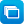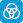Close message Scootle is changing in 2021.

# Search results

Year level
Resource type
Learning area

## Refine by topic

Main topic Specific topic Related topic
Listed under:  Data analysis### Data - Years 7 to 9

The 12 learning objects in this collection relate to the use, collection, display, comparison and interpretation of data. Objects in the first category, statistical data graphs, enable students to explore data using a range of graphical analysis tools. Objects in the second category, analysing surveys, enable students to ...### Fix the matchbox machine: scoop size

Check whether a machine is packing most matchboxes with an acceptable number of matches (40–60 matches per box). Look at boxplots made after taking samples of 100 matchboxes. Analyse the data and identify whether results are within the tolerance range. Adjust the machine's scoop size (if needed) to make it work correctly. ...### Fix the matchbox machine: speed

Check whether a machine is packing most matchboxes with an acceptable number of matches (40–60 matches per box). Look at boxplots made after taking samples of 100 matchboxes. Analyse the data and identify whether results are within the tolerance range. Adjust the machine's scoop size (if needed) to make it work correctly. ...### Matchbox machine: take a sample

Check whether a machine is packing most matchboxes with an acceptable number of matches (40–60 matches per box). Take a sample of 100 matchboxes and make a boxplot to analyse the results. Sort the sample data into four equal groups. Identify the range, median, first and third quartile values. Position the data points to ...### Matchbox machine: plot the variation

Check whether a machine is packing most matchboxes with an acceptable number of matches (40–60 matches per box). Take a sample of 100 matchboxes and make a boxplot to analyse the results. Sort the sample data into four equal groups. Identify the range, median, first and third quartile values. Position the data points to ...### Matchbox machine

Check whether a machine is packing most matchboxes with an acceptable number of matches (40–60 matches per box). Take a sample of 100 matchboxes and make a boxplot to analyse the results. Sort the sample data into four equal groups. Identify the range, median, first and third quartile values. Position the data points to ...### Rice crisp machine

Check whether a machine is packing most bags with an acceptable number of rice crisps (20–35 rice crisps per packet). Take a sample of 100 packets and make a boxplot to analyse the results. Sort the sample data into four equal groups. Identify the range, median, first and third quartile values. Position the data points ...### Rice crisp machine: take a sample

Check whether a machine is packing most bags with an acceptable number of rice crisps (20–35 rice crisps per packet). Take a sample of 100 packets and make a boxplot to analyse the results. Sort the sample data into four equal groups. Identify the range, median, first and third quartile values. Position the data points ...### Fix the matchbox machine: scoop size and speed

Check whether a machine is packing most matchboxes with an acceptable number of matches (40–60 matches per box). Look at boxplots made after taking samples of 100 matchboxes. Analyse the data and identify whether results are within the tolerance range. Adjust the machine's scoop size or speed (if needed) to make it work ...### Rice crisp machine: plot the variation

Check whether a machine is packing most bags with an acceptable number of rice crisps (20–35 rice crisps per packet). Take a sample of 100 packets and make a boxplot to analyse the results. Sort the sample data into four equal groups. Identify the range, median, first and third quartile values. Position the data points ...### Scatter plots

Find out what a scatter plot is and how it can be used to investigate relationships between two variables. Analyse data for a pair of variables such as hand span and foot length. Enter your personal data and plot it with other data on a graph. Use a line of best fit to identify if there is a positive or negative relationship ...### Scatter plots: height and bellybutton height

Find out what a scatter plot is and how it can be used to investigate relationships between two variables. Analyse data for a pair of variables: a person's height and the height of their bellybutton measured from the floor. Enter your personal data and plot it with other data on a graph. Use a line of best fit to identify ...### Scatter plots: age and reaction time

Find out what a scatter plot is and how it can be used to investigate relationships between two variables. Analyse data for a pair of variables: a person's age and the time it takes them to react to a signal. Enter your personal data and plot it with other data on a graph. Use a line of best fit to identify if there is ...### Scatter plots: foot length and hand span

Find out what a scatter plot is and how it can be used to investigate relationships between two variables. Analyse data for a pair of variables: hand span and foot length. Enter your personal data and plot it with other data on a graph. Use a line of best fit to identify if there is a positive or negative relationship between ...### Scatter plots: create your own data

Find out what a scatter plot is and how it can be used to investigate relationships between two variables. Gather and analyse data for a pair of variables such as hand span and foot length. Enter your data set and plot it on a graph. Use a line of best fit to identify if there is a positive or negative relationship between ...### Data - Years 5 and 6

The 11 learning objects in this collection relate to the use, collection, display, comparison and interpretation of statistical data. Objects in the first category, statistical graphs, enable students to compare and use a broad range of statistical graphs appropriately. Objects in the second category, surveys, explore and ...### Australia's population: countries of birth - dataset

The dataset provides statistics about the estimated resident population, median age and sex ratio by countries of birth for the latest year of available data. It is periodically updated by the Australian Bureau of Statistics (ABS). The dataset is included in the list of related datasets on the page in MS Excel format.### Mean, median and mode

This resource is a video demonstration, with audio commentary, about calculating the mean, median and mode of a data set. The meaning of each of the terms - mean, median and mode - is explained and the difference between them is clarified. The resource explains the process and demonstrates a handwritten method for calculating ...### Inference for proportions

This is a teacher resource for inference of proportions consisting of a website and a PDF with identical content. It contains discussion of how probability is used in statistical inference, the sample proportion as an estimator, the sample proportion as a random variable, population parameters and sample estimates, and ...### Using data about favourite foods

Flynn helps Dodly gather information about monsters' favourite foods for Dodly's new cafe menu. Watch and learn while the two monsters research their friends' favourite foods and then display and organise this information. Will Flynn ever get fed at Cafe de Dodly?